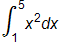The following strategies for answering the free-response questions will help you on exam day.

## Keep an eye on your time.

Monitor your time carefully. Make sure not to spend too much time on any one question so you’ll have enough time to answer all of them. If you do work that you think is incorrect, simply put an “X” through it instead of spending time erasing it completely: crossed-out work won’t be graded.

## Show your work, even when you’re using a calculator.

Show all the steps you took to reach your solution on questions involving calculations. The exam reader wants to see if you know how to solve the problem. If you use your calculator to solve an equation, compute a numerical derivative, or find a definite integral, then be sure to write the equation, derivative, or integral first: an answer without this information might not get full credit, even if the answer is correct. Remember to write your work in standard notation (e.g.) rather than calculator syntax (e.g. fnInt(X2,X,1,5), as calculator syntax is not acceptable.

## Try to solve each part of the question.

Many free-response questions are divided into parts such as (a), (b), (c), and (d), with each part calling for a different response. Credit for each part is awarded independently, so you should attempt to solve each part. For example, you may receive no credit for your answer to part (a), but still receive full credit for part (b), (c), or (d). If the answer to a later part of a question depends on the answer to an earlier part, you may still be able to receive full credit for the later part, even if that earlier answer is wrong.

## Be sure to fully answer the question being asked.

For example, if a question asks for the maximum value of a function, do not stop after finding the x-value at which the maximum value occurs. Be sure to express your answer in correct units if units are given and always provide a justification when it is asked for.

## When asked to justify or to explain an answer, think about how that can be done.

For example, if you are asked to justify a point of inflection, you need to show that the sign of the second derivative changes. Simply saying that the second derivative equals zero or is undefined is not a justification.

## Do not round partial answers.

Store partial answers in your calculator so that you can use them unrounded in further calculations.

## Practice!

Answering free-response questions from previous AP Exams is a great way to practice: it allows you to compare your own responses with those that have already been evaluated and scored. Free-response questions and scoring guidelines are available on the Exam Practice page for AP Calculus AB.